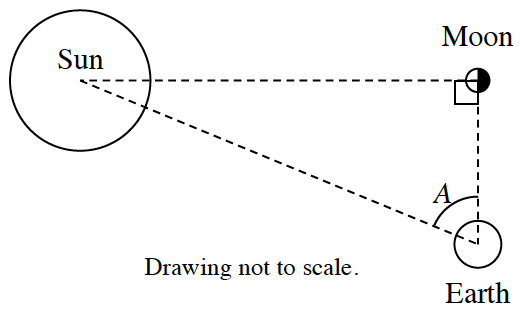### Home > CCG > Chapter 11 > Lesson 11.2.1 > Problem11-84

11-84.

The moon is an average distance of $238\text{,}900$ miles away from the Earth. While that seems very far, how far is it?

1. Compare that distance with the circumference of the Earth’s equator. Assume that the Earth’s radius is $4000$ miles. How many times greater than the Earth’s circumference is the distance to the moon?

The formula to find the circumference of a circle is $2πr$.

$≈ 9.5$ times greater

1.One way to estimate the distance between the Earth and the sun is to consider the triangle formed by the sun, Earth, and moon when the moon appears to be half-full. (See the diagram at right.) When the moon appears from earth to be half-full, it can be assumed that the moon forms a $90º$ angle with the sun and the Earth.

Using special equipment, Ray found the measure of angle $A$ to be $89.85º$. If the moon is $238\text{,}900$ miles away from the Earth, then how far is the sun from the Earth?

$\text{cos }\angle A =\frac{\text{distance between Earth and Moon}}{\text{distance between Earth and Sun}}$

$≈ 91\text{,}253\text{,}182.4$ miles